#Software Freedom Celebration

SMVDU## Speaker

Parbhat Puri (@parbhat)
Nick: appa
parbhatpuri17@gmail.com
http://parbhat.github.io/
http://www.github.com/parbhat

Software Freedom Day is a worldwide celebration of Free and Open Source Software (FOSS). Our goal in this celebration is to educate you about the benefits of using high quality FOSS in education, in government, at home, and in business -- in short, everywhere!

What is Open Source ?

What is Python ?

## This is Python....## History

`Over six years ago, in December 1989, I was looking for a "hobby" programming project that would keep me occupied during the week around Christmas. My office ... would be closed, but I had a home computer, and not much else on my hands. I decided to write an interpreter for the new scripting language I had been thinking about lately: a descendant of ABC that would appeal to Unix/C hackers. I chose Python as a working title for the project, being in a slightly irreverent mood (and a big fan of Monty Python's Flying Circus).`
• Salute this guy : Guino Van Rossum
• Python conceived in late 1980s
• Implementation was started in Dec 1989## WHAT IS PYTHON?

• A dynamic, interpreted, high-level language
• Does not require declaring the types of variables or parameters or methods
• Used for solving a really wide range of problems
• Object-oriented
• Does not force Object-orientation
• Has and requires common sense

## Who all are using Python?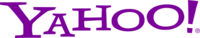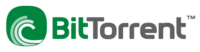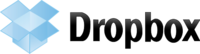and the list goes on...

## Why Python?

• Used by many companies across the world including Google, Rackspace, Industrial Light and Magic, Flickr and many others.
• A thriving community of developers and contributors and third party application developers.
• PyPI (Python Package Index) lists about 33500 third-party software projects*... You name an area, there must be a Python package in it and mostly opensource and free!
• Interpreted, dynamically typed, clean syntax, very easy to learn.
• Learn Python in an Afternoon ;) - http://docs.python.org/2/tutorial/

#### Where ?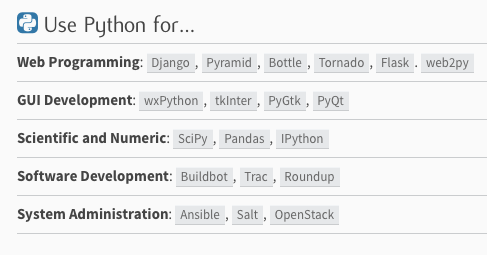## Show me the Money ?

• IT salaries are up
• Python is top growing skills last year
• Average starting python programmer salary 440K INR

## How to start ?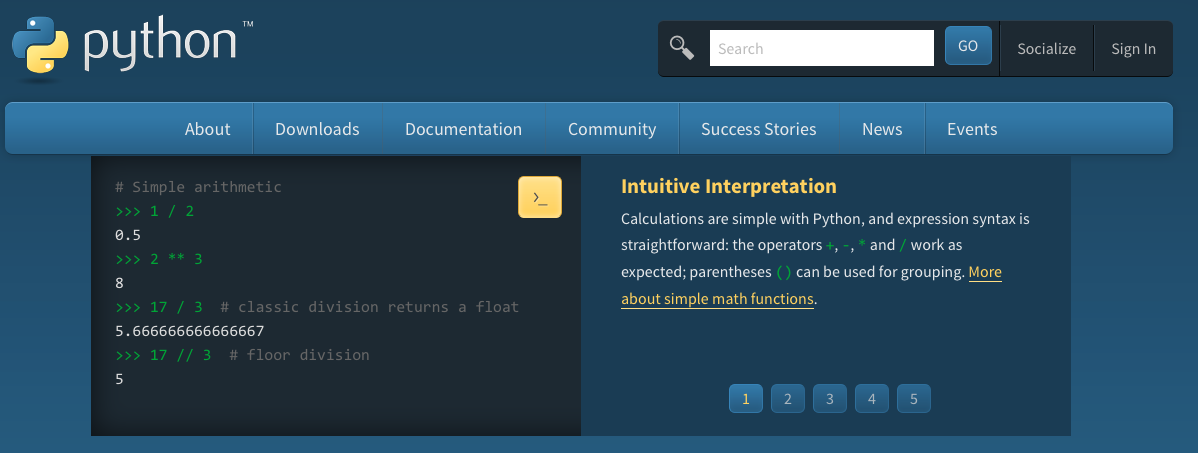## How it's done with Java and C++

``````public class HelloWorld {
public static void main(String[] args) {
System.out.println("Hello, World");
}
} ``````

``````#include
main()
{
cout << "Hello World!";
return 0;
} ``````

Requires a LOT more than just common sense.

## The Pythonic way

Common sense: When you want to print a string, just print it.

In Python interpreter
`` >>> print "Hello, world."``

As a script
Open your favorite editor and type
``````#! /usr/bin/env python
print "Hello, world."``````
and save it as hello.py. And then execute it from command line as below
``\$ python hello.py``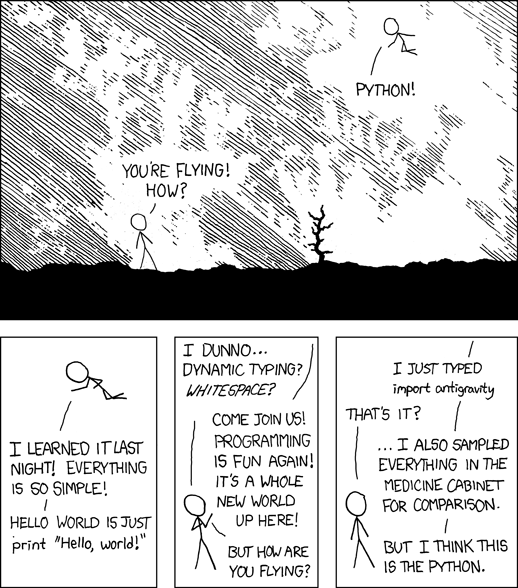## VARIABLES & DATATYPES

• Identifiers
• Keywords
• Assignments
• Operators  & Expressions

## IDENTIFIERS

``identifier ::= (letter|"_") (letter | digit | "_")*``letter ::= lowercase | uppercase``lowercase ::= "a"..."z"``uppercase ::= "A"..."Z"``digit ::= "0"..."9"``

So, while abc is a valid identifier, 1abc is not :)

## KEYWORDS

``and        del       from      not     while``as         elif      global    or      with``assert     else      if        pass    yield``break      except    import    print``class      exec      in        raise``continue   finally   is        return``def        for       lambda    try``

## Assignments

In Python, we don't declare what kind of data we want to put in a variable.
`` >>> a = 12`` >>> b = 23`` >>> a + b`` >>> 35``

Examples
Multiple assignments

``>>> a, b  = 10, 12``>>> print a``10``>>> print b``12``

## Python is fun

Let's try to swap 2 numbers
``# The usual way``>>> a = 10``>>> b = 20``>>> c = b``>>> b = a``>>> a = c``>>> print a, b``20 10``
``# The Pythonic way``>>> a, b = 10, 20``>>> a, b = b, a``>>> print a, b``20 10``

## DATATYPES

``# Integer``>>> a = 10``# Float``>>> b = 2.35``# String``>>> c = "Hello"``# List``>>> d = [1, 2, 'blah', 3.2]``# Dictionary``>>> f = {'a': 1, 'b': 2.3, 'c': "foo"}``>>> f['b']``2.3``

## Operators

• Arithmetic: +, -, /, *, %, //
• Relational: >, <, >=, <=, ==, !=
• Logical: and, or
• Shorthand: +=, /=, -=, *=

## Arithmetic operators

``````>>> 2 + 3
5
>>> 23 - 3
20
>>> 22.0 / 12
1.8333333333333333````>>> 14 % 3``2````>>> 4.0 // 3
1.0
>>> 4.0 / 3
1.3333333333333333``````

## Logical operators

To do logical AND , OR we use and ,*or* keywords. x and y returns False if x is False else it returns evaluation of y. If x is True, it returns True.

``````>>> 1 and 4
4
>>> 1 or 4
1
>>> -1 or 4
-1
>>> 0 or 4
4 ``````

## Shorthand Operators

x op = expression is the syntax for shorthand operators. It will be evaluated like x = x op expression , Few examples are

``````>>> a = 12
>>> a += 13
>>> a
25
>>> a /= 3
>>> a
8
>>> a += (26 * 32)
>>> a
840 ``````

## Expressions

Expressions are made of operators and operands. An expression is like 2 + 3 .
Generally while writing expressions we put spaces before and after every operator so that the code becomes clearer to read, like
``a = 234 * (45 - 56.0 / 34) ``
How expressions are evaluated
`(6 + 3) - 12 / 3 + 3 * 2 -19 - 12 / 3 + 3 * 2 -19 - 4 + 3 * 2 - 19 - 4 + 6 - 15 + 6 - 111 - 110`

## Type Conversions

• float(string) -> float value
• int(string) -> integer value
• str(integer) or str(float) -> string representation

``````>>> a = 8.126768
>>> str(a)
'8.126768' ``````

## If-else , the control flow

``````if expr1:
do this
elif expr2:
do that
...
...
...
else:
do whatever ``````
Truth value testing
DO
``````if x:
pass ``````
DON'T
``````if x == True:
pass``````

``` ```

# Functions

## Defining a function

``````def functionname(params):
statement1
statement2 ``````

## Sum of 2 integers

>>> def sum(a, b):
...     return a + b
>>> res = sum(234234, 34453546464)
>>> res
34453780698L

## Local & global variables

``````#!/usr/bin/env python
def change(b):
a = 90
print a
a = 9
print "Before the function call ", a
print "inside change function",
change(a)
print "After the function call ", a ``````

The output
``````\$ ./local.py
Before the function call  9
inside change function 90
After the function call  9 ``````

An example for global variable usage
``````#!/usr/bin/env python
def change(b):
global a
a = 90
print a
a = 9
print "Before the function call ", a
print "inside change function",
change(a)
print "After the function call ", a ``````

## Default argument value

``````>>> def test(a , b=-99):
...     if a > b:
...         return True
...     else:
...         return False ``````

``````>>> test(12, 23)
False
>>> test(12)
True ``````

## Keyword arguments

``````>>> def func(a, b=5, c=10):
...     print 'a is', a, 'and b is', b, 'and c is', c
...
>>> func(12, 24)
a is 12 and b is 24 and c is 10
>>> func(12, c = 24)
a is 12 and b is 5 and c is 24
>>> func(b=12, c = 24, a = -1)
a is -1 and b is 12 and c is 24 ``````

Guess the output of this ;)
``````>>> def func(a, b=13, v):
...     print a, b, v
... ``````

# Lists

``>>> a = [23, 45, 1, -3434, 43624356, 234] ``
``````# Append
>>> a.append(45)``````
``````>>> a
[23, 45, 1, -3434, 43624356, 234, 45] ``````
``````# Insert
>>> a.insert(0, 1) # 1 added at the 0th position of the list``````
``````>>> a
[1, 23, 45, 1, -3434, 43624356, 234, 45] ``````
``>>> a.insert(0, 111)``
``````>>> a
[111, 1, 23, 45, 1, -3434, 43624356, 234, 45]``````
``````# Count the length of the list
>>> a.count(45)
2 ``````

``````# Remove a element from the list
>>> a.remove(234) ``````
``````>>> a
[111, 1, 23, 45, 1, -3434, 43624356, 45] ``````
``````# Reverse a list
>>> a.reverse() ``````
``````>>> a
[45, 43624356, -3434, 1, 45, 23, 1, 111] ``````
``````# Append a list into another list
>>> b = [45, 56, 90]
>>> a.append(b) ``````
``````>>> a
[45, 43624356, -3434, 1, 45, 23, 1, 111, [45, 56, 90]]
>>> a[-1]
[45, 56, 90] ``````
``````# Extend a into b
>>> a.extend(b) #To add the values of b not the b itself
>>> a
[45, 43624356, -3434, 1, 45, 23, 1, 111, [45, 56, 90], 45, 56, 90]
>>> a[-1]
90 ``````
``````# Sort a list
>>> a.sort()
>>> a
[-3434, 1, 1, 23, 45, 45, 45, 56, 90, 111, 43624356, [45, 56, 90]] ``````
``````# Delete a element in list
>>> del a[-1]
>>> a
[-3434, 1, 1, 23, 45, 45, 45, 56, 90, 111, 43624356] ``````
``````# Basic Data Structure
>>> a = [1, 2, 3, 4, 5]
>>> a.append(1)
>>> a
[1, 2, 3, 4, 5, 1]``````
``````>>> a.pop(0)
1
>>> a.pop(0)
2
>>> a
[3, 4, 5, 1] ``````

## List Comprehensions

``````# List Comprehensions
>>> a = [1, 2, 3]
>>> [x ** 2 for x in a]
[1, 4, 9]
>>> z = [x + 1 for x in [x ** 2 for x in a]]
>>> z
[2, 5, 10]``````

# Looping

## While Loop

``````while condition:
statement1
statement2 ``````
````>>> n = 0````>>> while n < 11:
...     print n
...     n += 1
...
0
1
2
3
4
5
6
7
8
9
10 ``````

## For Loop

``>>> a = ['Python', 'is', 'powerful'] ``

``````>>> for x in a:
...     print x,
...
Python is powerful ``````

``````>>> range(1, 5)
[1, 2, 3, 4]``````
``````>>> range(1, 15, 3)
[1, 4, 7, 10, 13]``````
``````>>> range(10)
[0, 1, 2, 3, 4, 5, 6, 7, 8, 9]``````
``````>>> range(10, 1, -2)
[10, 8, 6, 4, 2]``````

## For...else...

``````>>> for i in range(0, 5):
...     print i
... else:
...     print "Bye bye"
...
0
1
2
3
4
Bye bye``````

# Sets

``````# Set: Another data-structure with no duplicate items
>>> a = set('abcthabcjwethddda')
>>> a
set(['a', 'c', 'b', 'e', 'd', 'h', 'j', 't', 'w']) ``````
``````# Set Operations
>>> b = set('alacazam')``````
``````>>> a                                  # unique letters in a
set(['a', 'r', 'b', 'c', 'd'])``````
``````>>> a - b                              # letters in a but not in b
set(['r', 'd', 'b'])``````
``````>>> a | b                              # letters in either a or b
set(['a', 'c', 'r', 'd', 'b', 'm', 'z', 'l'])``````
``````>>> a & b                              # letters in both a and b
set(['a', 'c']) ``````
``````>>> a ^ b                              # letters in a or b but not both
set(['r', 'd', 'b', 'm', 'z', 'l']) ``````
``````>>> a
set(['a', 'c', 'b', 'e', 'd', 'h', 'j', 'q', 't', 'w'])
>>> a
set(['a', 'c', 'b', 'e', 'd', 'h', 'j', 'q', 'p', 't', 'w']) ``````

## Strings

``````>>> s = "I am Indian"
>>> s
'I am Indian'
>>> s = 'I am Indian'
>>> s = "Here is a line \
... splitted in two lines"
>>> s
'Here is a line split in two lines'
>>> s = "Here is a line \n split in two lines"
>>> s
'Here is a line \n split in two lines'
>>> print s
Here is a line
split in two lines ``````
``````>>> s = """ This is a
... multiline string, so you can
... write many lines"""
>>> print s
This is a
multiline string, so you can
write many lines ``````

``````# String Methods
>>> s = "We all love Python" ``````
``````>>> s.split(" ")
['We', 'all', 'love', 'Python']``````
``````>>> x = "Nishant:is:waiting"
>>> x.split(':')
['Nishant', 'is', 'waiting']``````
``````>>> "-".join("GNU/Linux is great".split(" "))
'GNU/Linux-is-great' ``````
``````>>> s = "  abc\n "
>>> s.strip()
'abc' ``````
``>>> s = "faulty for a reason"``
``````>>> s.find("for")
7``````
``````>>> s.find("fora")
-1 ``````

``>>> s.startswith("fa") #To check if the string startswith fa or not True``>>> s.endswith("reason") # True ``
``````>>> s = "sayan chowdhury"
>>> s.title()
'Sayan Chowdhury' ``````
``````>>> z = s.upper()
>>> z
'SAYAN CHOWDHURY'
>>> z.lower()
'sayan chowdhury'``````
``````>>> s = "I am A pRoGraMMer"
>> s.swapcase()
'i AM a PrOgRAmmER'``````

## File-Handling

``>>> f = open('foo.txt', 'w') ``
``>>> f.write("First line") ``
``>>> f.close() ``
``>>> f = open('foo.txt', 'r') ``
``````>>> f.readline()
'First line' ``````
``````>>> f.readline()
'' ``````
``>>> f = open('foo.txt', 'a') ``
``>>> f.write('Second line') ``
``>>> f.close() ``
``>>> f = open('foo.txt', 'r') ``
``````>>> f.readline()
'First lineSecond line'``````

``>>> f = open('foo.txt', 'a') ``
``>>> f.write("New line\n") ``
``````>>> f.write("One more new line")
>>> f.close()        ``````
``>>> f = open('foo.txt', 'r') ``
``````>>> f.readline()
'First lineSecond lineNew line\n' ``````
``````>>> f.readline()
'One more new line'
''``````
``>>> f.close() ``
``>>> f = open('foo.txt') ``
``````>>> f.readlines()
['First lineSecond lineNew line\n', 'One more new line'] ``````

``>>> f = open('foo.txt', 'w') ``
``````>>> f.writelines(["1\n", "2\n"])
>>> f.close() ``````
``>>> f = open('foo.txt') ``
``````>>> f.readlines()
['1\n', '2\n'] ``````
``>>> f.close()``

## An example

``````"""
Bars Module
============
This is an example module with provide different ways to print bars.
"""
def starbar(num):
"""
Prints a bar with *
:arg num: Length of the bar
"""
print '*' * num

def hashbar(num):
"""
Prints a bar with #
:arg num: Length of the bar
"""
print '#' * num

def simplebar(num):
"""
Prints a bar with -
:arg num: Length of the bar
"""
print '-' * num ``````

``>>> import bars``>>> bars.hashbar(10)``##########``

## Importing modules

``````>>> from bars import simplebar, starbar
>>> simplebar(10)
>>> ----------
````# NEVER DO THIS``>>> from bars import *````

## Submodules

We can have many submodules inside a module.

``````mymodule
|-- bars.py
|-- __init__.py
`-- utils.py ``````

## Default modules

help:
``>>> help(str) ``
os:
``````>>> import os
>>> os.getpid()
14875
>>> os.getcwd()
'/Users/rtnpro'
>>> os.chdir('/tmp')
>>> os.getcwd()
'/tmp' ``````

## Example

``````def view_dir(path='.'):
"""
This function prints all files and directories in the given directory.
:args path: Path to the directory, default is current directory
"""
names = os.listdir(path)
names.sort()
for name in names:
print name, ``````
Using the view_dir() example:
``````>>> view_dir('/')
.readahead bin boot dev etc home junk lib lib64 lost+found media mnt opt
proc root run sbin srv sys tmp usr var ``````

## Fun exercise

Are you ready for the challenge?

## Solution

``````from BeautifulSoup import BeautifulSoup
import requests

url = 'http://pyvideo.org/category/33/pycon-us-2013/files'
req = requests.get(url)
data = req.text
soup = BeautifulSoup(data)

#'http://s3.us.archive.org/nextdayvideo/psf/pycon2013/'):
'http://s3.us.archive.org/nextdayvideo/psf/pycon2013/'):

## Python fun

Check my university result
--> In just 32 lines of code

Friend result scrap by enrollment number
--> In just 20 lines of code

Ti zen application
--> In just 300 lines of code

Railway site check tickets booked
--> In just 70 lines of code

## Python Fun

Flipkart price change monitor
--> In just 55 lines of code

Friend want galaxy S near x amount
--> Wrote it in just 50 lines of code.

Product reviews
--> Wrote in just 150 lines of code

Get photos of my friends
--> Wrote it in just 80 lines of code

Merged bloggers
--> Wrote in just 70 lines of code

## Python Fun

Script to transfer file from pc to mobile
--> In just 1 line
python -m SimpleHTTPServer 8000

--> In just 5 line of code

Cricket live score
--> In just 6 lines of code

Flipkart check if my product came or not
--> In just 50 lines of code

Wanted 50-60 images to test gallery
--> In just 7 lines of code.

• http://pypi.python.org - Python Package Index
• http://docs.python.org/2/tutorial/ - The “official” Python tutorial
• http://wiki.python.org/moin/MostPopularPythonProjects -
• MostPopularPythonProjects
• http://www.python.org/community/workshops/ - Python
• Conferences and Workshops
• http://wiki.python.org/moin/ - The Python “MoinMoin” Wiki
• http://docs.python.org/devguide/ - All about developing Python
• http://mail.python.org/mailman/listinfo - A list of all Python
• http://pymbook.readthedocs.org/en/latest/ - Python for you & me

Thank You

Any questions ?

By Parbhat Puri

# SFD Python Workshop

Python Workshop organised in Software Freedom Day 2014.

• 1,825